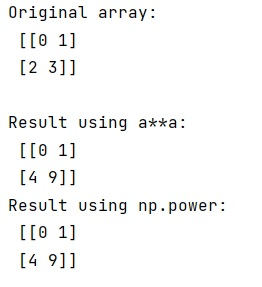# How to square or raise to a power (elementwise) a 2D numpy array?

Learn, how to square or raise to a power (elementwise) a 2D numpy array in Python?
Submitted by Pranit Sharma, on February 17, 2023

NumPy is an abbreviated form of Numerical Python. It is used for different types of scientific operations in python. Numpy is a vast library in python which is used for almost every kind of scientific or mathematical operation. It is itself an array which is a collection of various methods and functions for processing the arrays.

## Squaring or raising to a power (elementwise) a 2D numpy array

Suppose that we are given a 2D array and we need to square each of its elements.

There are a few notations with the help of which we can square the values of a numpy array like a**a, a**2, numpy.square(a), or numpy.power(a,2). However, numpy methods are shown to be slower but numpy.power() allows you to use different exponents for each element if instead of 2 you pass another array of exponents.

We can computer the square of each element using different notations. Let us understand with the help of an example,

## Python code to square or raise to a power (elementwise) a 2D numpy array

```# Import numpy
import numpy as np

# Creating numpy array
arr = np.arange(4).reshape(2, 2)

# Display original array
print("Original array:\n",arr,"\n")

# Squaring the array using a**a
res = arr*arr

# Display result
print("Result using a**a:\n",res)

# Squaring the array using np.power(a,2)
res = np.power(arr,2)

# Display result
print("Result using np.power:\n",res)
```

Output: